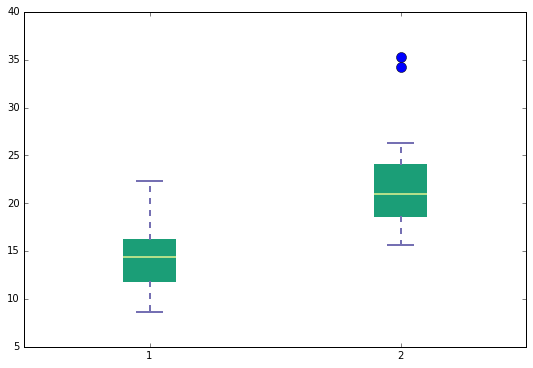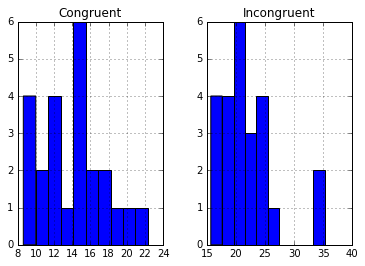Stroop Effect: Testing a Perceptual Phenomenon

In a Stroop task, participants are presented with a list of words, with each word displayed in a color of ink. The participant’s task is to say out loud the color of the ink in which the word is printed. The task has two conditions: a congruent words condition, and an incongruent words condition. In the congruent words condition, the words being displayed are color words whose names match the colors in which they are printed: for example RED, BLUE. In the incongruent words condition, the words displayed are color words whose names do not match the colors in which they are printed: for example PURPLE, ORANGE. In each case, we measure the time it takes to name the ink colors in equally-sized lists. Each participant will go through and record a time from each condition.

My test results:

Congruent words - 10.905

Incongruent words - 34.41

#### 1. What is our independent variable? What is our dependent variable?

Independent variable is: The word/colour congruency is the varable being manipulated in the experiment

Dependent variable is: The time it takes to recognize/name the ink colors of the mismatch word/colour congruency

#### 2. What is an appropriate set of hypotheses for this task? What kind of

statistical test do you expect to perform? Justify your choices. Null Hypothsis, H0 - The mismatch of color to word will have no effect or decrease time to recognize and say the color

Alternate Hypothesis, H1 - The mismatch of color to word will increase time to recognize and say the color

H0: μi ≤ μc (μi - population mean of incongruent values, μc - population mean of congruent values)

H1: μi > μc (μi - population mean of incongruent values, μc - population mean of congruent values)

statistical test choices and assumptions

• 95% confidence interval

• Paired one tail t-test -> with two tests per participant this test show if the mean of incongruent words is statistically significantly different from the congruent words at an alpha of 0.05.

assumptions/why: I will be using a t-test instead of a z-test because 1) the population standard deviation is unknown and 2) the sample set is less than 30. The t-test will be a one tailed t-test i.e. my directional alternative hypothesis is that participant’s incongruent sample mean will be larger than the participant’s congruent sample mean

A paired t-test (or dependent sample test), will be used because the data set is of one group of participants tested twice under different conditions (word/colour congruency). This will also facilitate either rejecting or accepting the null hypothesis.

#### 3. Report some descriptive statistics regarding this dataset. Include at

least one measure of central tendency and at least one measure of variability.

sample size = 24

mean: $xbar = \Sigma{x}/n$ (where xbar is the sample mean, x is the value and n is the number of samples)

Congruent: 14.05, Incogruent: 22.02

median: as the data seems slightly positively skewed, median is a better representation of central tendency

Congruent: 14.3565, Incongruent: 21.0175

sample std. deviation: $\sigma = \Sigma{(x - xbar)^2}/n$

Congruent: 3.56, Incongruent: 4.80

In :

In :

    Congruent  Incongruent
0      12.079       19.278
1      16.791       18.741
2       9.564       21.214
3       8.630       15.687
4      14.669       22.803
5      12.238       20.878
6      14.692       24.572
7       8.987       17.394
8       9.401       20.762
9      14.480       26.282
10     22.328       24.524
11     15.298       18.644
12     15.073       17.510
13     16.929       20.330
14     18.200       35.255
15     12.130       22.158
16     18.495       25.139
17     10.639       20.429
18     11.344       17.425
19     12.369       34.288
20     12.944       23.894
21     14.233       17.960
22     19.710       22.058
23     16.004       21.157


In :

Congruent Incongruent
count 24.000000 24.000000
mean 14.051125 22.015917
std 3.559358 4.797057
min 8.630000 15.687000
25% 11.895250 18.716750
50% 14.356500 21.017500
75% 16.200750 24.051500
max 22.328000 35.255000

In :

(14.3565, 21.0175)


#### 4. Provide one or two visualizations that show the distribution of the

sample data. Write one or two sentences noting what you observe about the plot or plots.

Plots

Please see below a boxplot and histogram which show the distribution of data from both congruent and incongruent conditions.

Observations

From the boxplot, there are two somewhat obvious outliers or extraneous data which would possible skew the true mean of incongruent values. And from the histogram plots, although both graphs visually appear somewhat positively skewed, the mean is pretty close to the peak in both graphs which would indicate a normal distribution. Provided these are samples from the population, the sampling mean would be similar to the population mean.

In :

array([[<matplotlib.axes._subplots.AxesSubplot object at 0x11e7c3b50>,
<matplotlib.axes._subplots.AxesSubplot object at 0x11e684b10>]], dtype=object)#### 5. Now, perform the statistical test and report your results. What is your

confidence level and your critical statistic value? Do you reject the null hypothesis or fail to reject it? Come to a conclusion in terms of the experiment task. Did the results match up with your expectations?

mean difference, dbar = $\Sigma (yi − xc)/n$ = 7.964

yi is incongruent, xc is the congruent values, n is the sample set

standard deviation, sd = 4.86

standard error of the difference, SE(dbar) = sd/√n = 4.86/√24 = 0.99

t-statistic, T = dbar/SE(dbar) = 7.964/0.99 = 8.04 on 23df

t-distribution with n-1 degrees of freedom (df = 23). Using the t-distribution table to find p-value…

The value of p is < 0.0001. The result is significant at p < 0.05%

Hypothesis

I reject the null hypothesis, the word/colour incongruent does cause a greater time response

Conclusion

The results match my expectations.

In :

    Congruent  InCongruent  Difference  Mean Difference  Std Deviation
0      12.079       19.278       7.199         7.964792       4.864827
1      16.791       18.741       1.950              NaN            NaN
2       9.564       21.214      11.650              NaN            NaN
3       8.630       15.687       7.057              NaN            NaN
4      14.669       22.803       8.134              NaN            NaN
5      12.238       20.878       8.640              NaN            NaN
6      14.692       24.572       9.880              NaN            NaN
7       8.987       17.394       8.407              NaN            NaN
8       9.401       20.762      11.361              NaN            NaN
9      14.480       26.282      11.802              NaN            NaN
10     22.328       24.524       2.196              NaN            NaN
11     15.298       18.644       3.346              NaN            NaN
12     15.073       17.510       2.437              NaN            NaN
13     16.929       20.330       3.401              NaN            NaN
14     18.200       35.255      17.055              NaN            NaN
15     12.130       22.158      10.028              NaN            NaN
16     18.495       25.139       6.644              NaN            NaN
17     10.639       20.429       9.790              NaN            NaN
18     11.344       17.425       6.081              NaN            NaN
19     12.369       34.288      21.919              NaN            NaN
20     12.944       23.894      10.950              NaN            NaN
21     14.233       17.960       3.727              NaN            NaN
22     19.710       22.058       2.348              NaN            NaN
23     16.004       21.157       5.153              NaN            NaN


#### 6. Optional: What do you think is responsible for the effects observed? Can

you think of an alternative or similar task that would result in a similar effect? Some research about the problem will be helpful for thinking about these two questions!

I believe it’s a subconscious or habitual behaviour where commonly used words are glanced over and easily recognized in your mind and therefore, because the color and words match, it takes little effort to say the word/colour. However, when the word and colour are mismatched, we first think of the word and then need to correct ourselves to say the colour causing either errors or more time to provide the correct response.

I imagine replacing letters with numbers in the experiment would yeild the same results.

Another similar task: being shown a list of words and asking the user to type (on a qwerty keyboard) commonly used words. First, use a list words with no spelling mistakes and then the same list of words with obviously misspelling or a number in the middle of the word. The reflects of knowing where the keys are positioned and the knoweledge of the correctly spelt word would allow cause the users to produce a fast time for correctly spelt words and a longer time to recognize that the word is spelt incorrectly and having to change their habitual behaviour, causing a lag and longer time to complet the word.

https://en.wikipedia.org/wiki/Stroop_effect

http://www.statisticshowto.com/when-to-use-a-t-score-vs-z-score/

http://www.biostathandbook.com/testchoice.html

http://www.ats.ucla.edu/stat/stata/whatstat/whatstat.htm